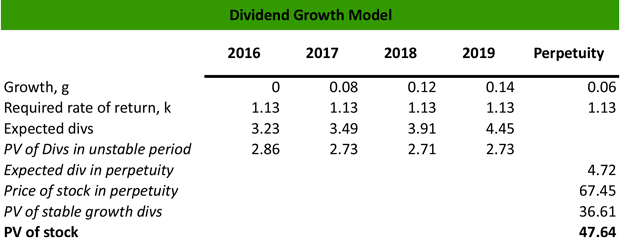# What is a Dividend Growth Model?

Definition: Dividend growth model is a valuation model, that calculates the fair value of stock, assuming that the dividends grow either at a stable rate in perpetuity or at a different rate during the period at hand.

## What Does Dividend Growth Model Mean?

What is the definition of dividend growth model? The dividend growth model determines if a stock is overvalued or undervalued assuming that the firm’s expected dividends grow at a value g forever, which is subtracted from the required rate of return (RRR) or k.

Therefore, the stable dividend growth model formula calculates the fair value of the stock as P = D1 / ( k – g ). The multistage stable dividend growth model equation assumes that g is not stable in perpetuity, but, after a certain point, the dividends are growing at a constant rate.

Let’s look at an example.

## Example

Company A is a leading retailer company that declares an annualized dividend of \$3.23 per share for 2017. Maria is a financial analyst who follows Company A, and she wants to calculate the fair value of the company stock using the dividend growth model.

Based on historical performance, Maria assumes that the company’s dividends will grow at a constant rate of 6% in perpetuity. She also assumes that the RRR based on the company’s fundamentals and historical data is 13%. Therefore, Maria calculates the price of the stock as follows:

P = D1 / ( k-g ) = \$3.23 / ( 13% – 6% ) = \$3.23 / 7% = \$46.14

The stock currently trades at \$54.68. Therefore, the stock is overvalued.

Maria wants to use the multistage dividend growth as well because assuming a constant dividend growth in perpetuity is not realistic.

Based on historical performance, Maria assumes that the company’s dividend will grow by 8% in 2017, 12% in 2018, 14% in 2019, and then will increase at a constant rate of 7%. The required rate of return is 13%.

Maria calculates the expected dividends from 2017 to 2019 based on the growth assumptions, and then for perpetuity. Then, she calculates the present value of expected dividends from 2017 to 2020 by raising the expected value to the power of 1, 2, 3 and so on. Therefore: 2016: \$3.23 / 1.13 = \$2.86, 2017: \$3.49 / 1.13^2 = \$2.73, 2018: \$3.91 / 1.13^3 = \$2.71 , 2019: \$4.45 / 1.13^4 = \$2.73.Then, she calculates the expected dividend for perpetuity = \$4.45 * ( 1 + 0.06 ) = \$4.72

Then, she calculates the fair value of the dividends for perpetuity = \$4.72 / (13% – 6%) = \$67.42

Therefore, the present value of the expected dividends from 2017 to 2019 = \$67.42 / ( 1.13 ) ^5 = \$36.61

The fair value of the stock = 2.86 + 2.73 + 2.71 + 2.73 + 36.61 = \$47.64

The stock currently trades at \$54.68. Therefore, the stock is overvalued.

## Summary Definition

Define Dividend Growth Model: DGM is a valuation method that investors use to determine an investment’s value by analyzing the dividend rate.